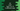# C# program to print from 1 to 10 in different ways### C# program to print from 1 to 10 by using a loop:

In this post, we will learn how to print from 1 to 10 by using a loop in C#. You can use the same methods to print from 1 to any number.

Loops are used to run a piece of code repeatedly. It runs for a specific number of times. Once the number is exceeded, it stops.

### C# program by using a for loop:

Let’s try to solve this by using a for loop. It looks as like below:

``````using System;
class Program {
static void Main() {
for(int i = 1; i <= 10; i++){
Console.WriteLine(i);
}
}
}``````

Here,

• Program is the class name. Main is the method that is called first when we run this program.
• We are using a for loop. This loop runs from i = 1 to i = 10. Inside the loop, we are printing the i values.

It will give output as like below:

``````1
2
3
4
5
6
7
8
9
10``````

### C# program by using a while loop:

We can also solve this by using a while loop. Let’s take a look at the program:

``````using System;
class Program {
static void Main() {
int i = 1;
while(i <= 10){
Console.WriteLine(i);
i++;
}
}
}``````

Here,

• We are using a while loop and it starts from i = 1.
• It runs till i = 10. For each value of i, it is printing the value of i. It is incrementing the value of i by 1 on each iteration.

It will print a similar output as the above program.

### Using a do-while loop:

We can also do this by using a do-while loop. do-while loop is similar to while loops. The only difference is that it runs the code inside do block and then checks for the condition in while. It looks as like below:

``````using System;
class Program {
static void Main() {
int i = 1;

do{
Console.WriteLine(i);
i++;
}while(i <= 10);
}
}``````

It will print a similar output.